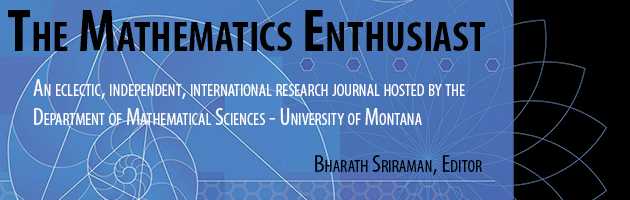•
•#### Article Title

Squaring the Circle and Doubling the Cube in Space-Time

18

1-2

#### Abstract

Squaring the Circle is a famous geometry problem going all the way back to the ancient Greeks. It is the great quest of constructing a square with the same area as a circle using a compass and straightedge in a finite number of steps. Since it was proven that ⇡ was a transcendental number in 1882, the task of Squaring the Circle has been considered impossible. Here, we will show it is possible to Square the Circle in space-time. It is not possible to Square the Circle in Euclidean space alone, but it is fully possible in space-time, and after all we live in a world with not only space, but also time. By drawing the circle from one reference frame and drawing the square from another reference frame, we can indeed Square the Circle. By taking into account space-time rather than just space, the impossible becomes possible! However, it is not enough simply to understand math in order to Square the Circle, one must understand some “basic” space-time physics as well. As a bonus, we have added a solution to the impossibility of Doubling the Cube. As a double bonus, we have also Boxed the Sphere! As we will see, one could claim that we have simply bent the rules and moved the problem from one place to another. One of the essential points of this paper, however, is that we can move challenging space problems out from space and into time, and vice versa.

59

77

#### Digital Object Identifier (DOI)

10.54870/1551-3440.1514

COinS